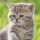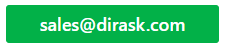Home
IT Knowledge
IT Career
Inspiration
Languages
EN

# JavaScript - e number approximation example

3 points
Created by:Kate_C
19800

The `Math.E` property returns `e` mathematical constant (`2.718281828459045...`).

`e` is called as Euler's number or as Napier's constant. However, it was discovered by the Jacob Bernoulli. It is a mathematical constant used as the base of the natural logarithm.

Using JavaScript it is possible to compute aproximation of `e` mathematical number in following way.

## 1. Infinity series example

To calculate `e` number folowing function with infinity series can be used - to get better precision infinite number of iterations with big precision numbers should be used.

``````// ONLINE-RUNNER:browser;

function computeE(iterations) {
var e = 0;

for (var i = 0; i < iterations; ++i) {
var divider = 1;

for (var j = 0; j < i; ++j) {
divider *= (j + 1);
}

e += (1 / divider);
}

return e;
}

console.log( computeE(  1 ) ); // 1
console.log( computeE(  2 ) ); // 2
console.log( computeE(  5 ) ); // 2.708333333333333
console.log( computeE( 10 ) ); // 2.7182815255731922
console.log( computeE( 20 ) ); // 2.7182818284590455
console.log( computeE( 50 ) ); // 2.7182818284590455``````

1. JavaScript - Math.E property example

## References

1. e (mathematical constant) - Wikipedia